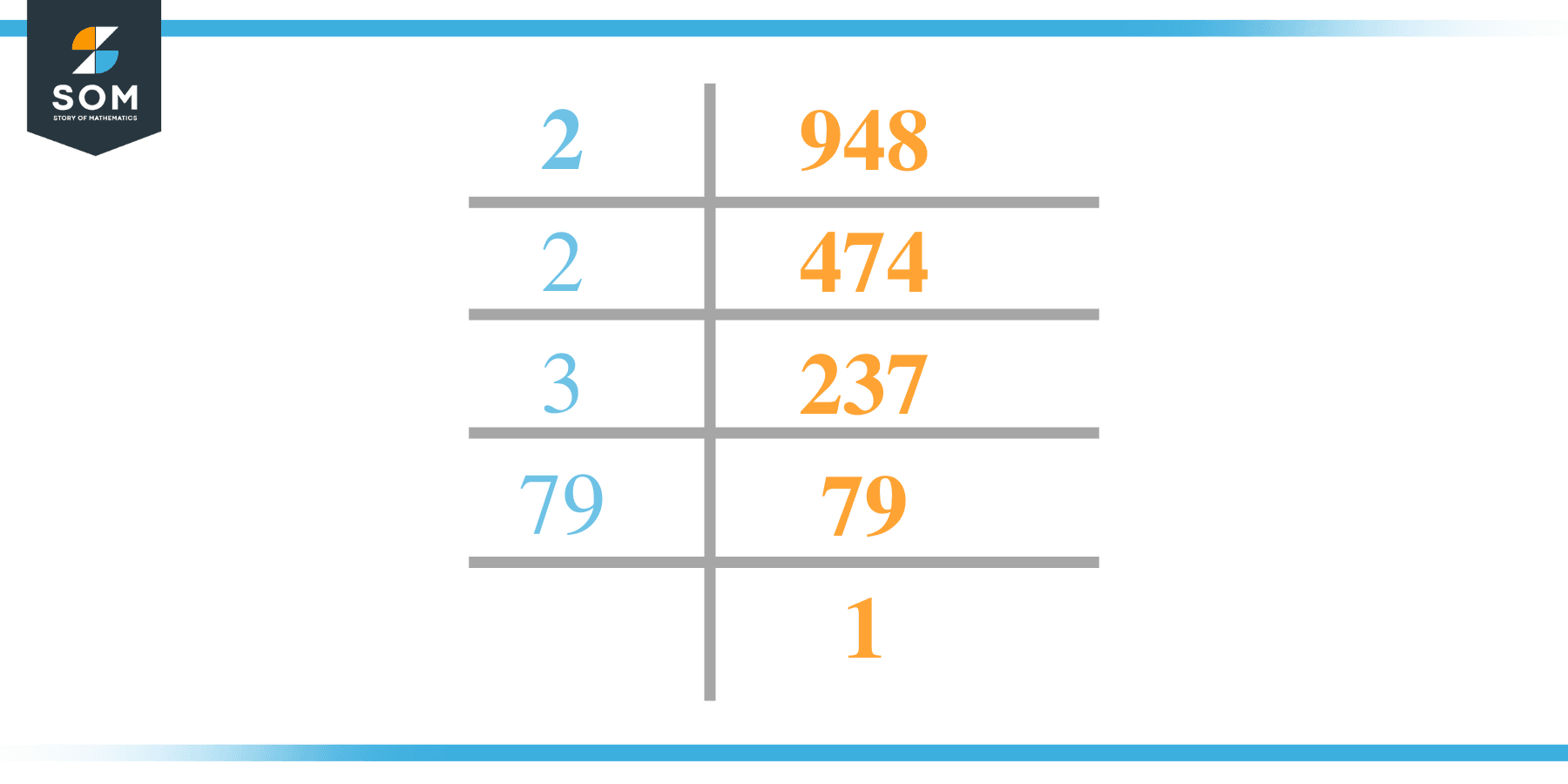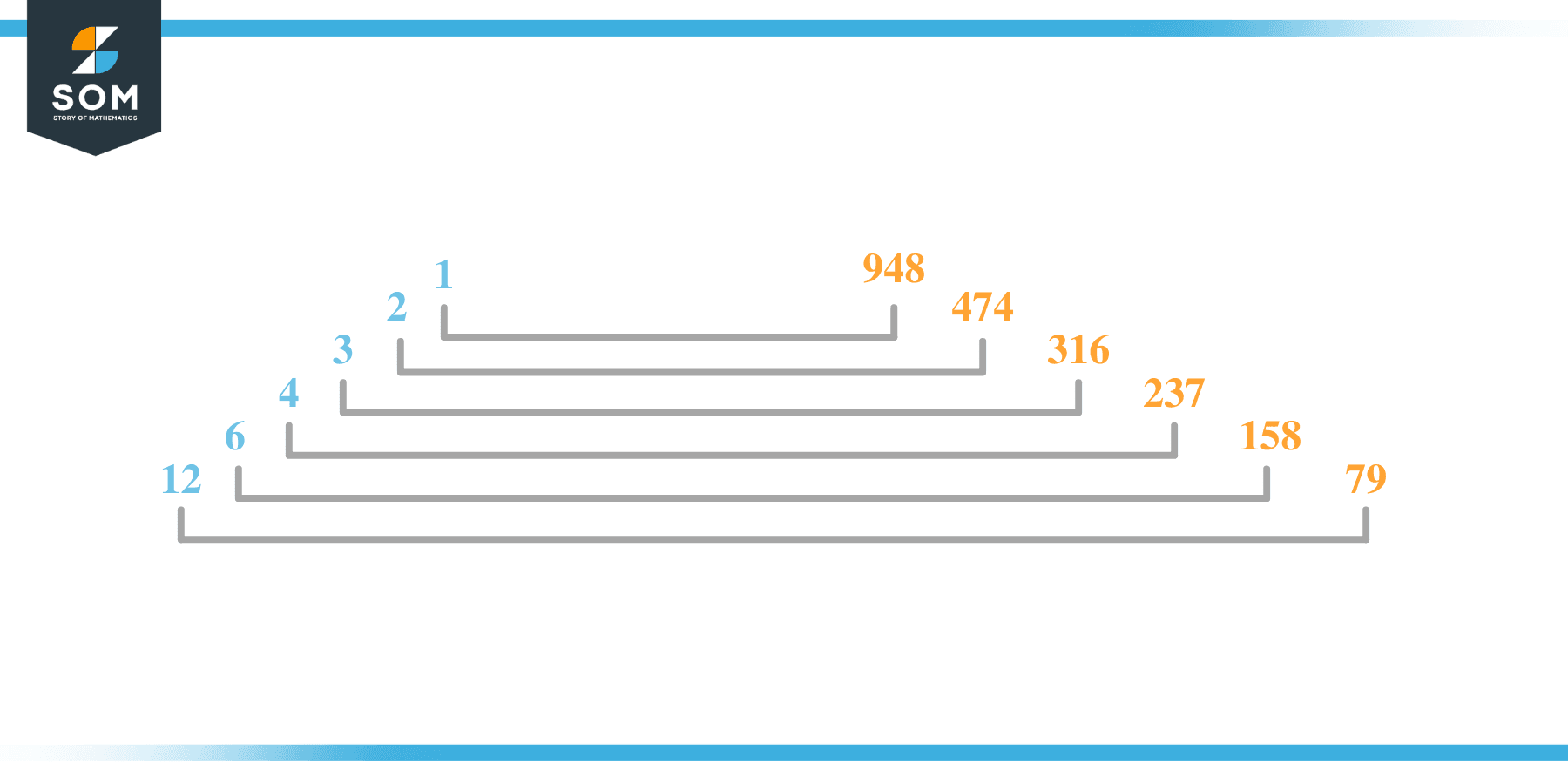# Factors of 948: Prime Factorization, Methods, and Examples

The factors of 948 are the listings of the integers that can evenly divide 948 into equal parts. There are twelve factors of 948, which indicates that all these twelve numbers are perfectly divisible by the number 948.Let us explore more about the factors.

### Factors of 948

Here are the factors of number 948.

Factors of 948: 1, 2, 3, 4, 6, 12, 79, 158, 237, 316, 474, and 948

### Negative Factors of 948

The negative factors of 948 are similar to their positive aspects, just with a negative sign.

Negative Factors of 948: –1, -2, -3, -4, -6, -12, -79, -158, -237, -316, -474, and -948

### Prime Factorization of 948

The prime factorization of 948 is the way of expressing its prime factors in the product form.

Prime Factorization: 2 x 2 x 3 x 79

In this article, we will learn about the factors of 948 and how to find them using various techniques such as upside-down division, prime factorization, and factor tree.

## What Are the Factors of 948?

The factors of 948 are 1, 2, 3, 4, 6, 12, 79, 158, 237, 316, 474, and 948. These numbers are the factors as they do not leave any remainder when divided by 948.

The factors of 948 are classified as prime numbers and composite numbers. The prime factors of the number 948 can be determined using the prime factorization technique.

## How To Find the Factors of 948?

You can find the factors of 948 by using the rules of divisibility. The divisibility rule states that any number, when divided by any other natural number, is said to be divisible by the number if the quotient is the whole number and the resulting remainder is zero.

To find the factors of 948, create a list containing the numbers that are exactly divisible by 948 with zero remainders. One important thing to note is that 1 and 948 are 948’s factors, as every natural number has 1 and the number itself as its factor.

1 is also called the universal factor of every number. The factors of 948 are determined as follows:

$\dfrac{948}{1} = 948$

$\dfrac{948}{2} = 474$

$\dfrac{948}{3} = 316$

$\dfrac{948}{4} = 237$

$\dfrac{948}{6} = 158$

$\dfrac{948}{12} = 79$

Therefore, 1, 2, 3, 4, 6, 12, 79, 158, 237, 316, 474, and 948 are the factors of 948.

### Total Number of Factors of 948

For 948, there are twelve positive factors and twelve negative ones. So in total, there are twenty-four factors of 948.

To find the total number of factors of the given number, follow the procedure mentioned below:

1. Find the factorization/prime factorization of the given number.
2. Demonstrate the prime factorization of the number in the form of exponent form.
3. Add 1 to each of the exponents of the prime factor.
4. Now, multiply the resulting exponents together. This obtained product is equivalent to the total number of factors of the given number.

By following this procedure, the total number of factors of 948 is given as:

The factorization of 948 is 1 x 2$^2$ x 3 x 79.

The exponent of 1, 3, and 79 is 1. The exponent of 2 is 2,

Adding 1 to each and multiplying them together results in twenty-four.

Therefore, the total number of factors of 948 is 24. Eight are positive, and eight factors are negative.

### Important Notes

Here are some essential points that must be considered while finding the factors of any given number:

• The factor of any given number must be a whole number.
• The factors of the number cannot be in the form of decimals or fractions.
• Factors can be positive as well as negative.
• Negative factors are the additive inverse of the positive factors of a given number.
• The factor of a number cannot be greater than that number.
• Every even number has 2 as its prime factor, the smallest prime factor.

## Factors of 948 by Prime FactorizationThe number 948 is a composite. Prime factorization is a valuable technique for finding the number’s prime factors and expressing the number as the product of its prime factors.

Before finding the factors of 948 using prime factorization, let us find out what prime factors are. Prime factors are the factors of any given number that are only divisible by 1 and themselves.

To start the prime factorization of 948, start dividing by its most minor prime factor. First, determine that the given number is either even or odd. If it is an even number, then 2 will be the smallest prime factor.

Continue splitting the quotient obtained until 1 is received as the quotient. The prime factorization of 948 can be expressed as:

948 = 2 x 2 x 3 x 79

## Factors of 948 in PairsThe factor pairs are the duplet of numbers that, when multiplied together, result in the factorized number. Factor pairs can be more than one depending on the total number of factors given.

For 948, the factor pairs can be found as:

1 x 948 = 948

2 x 474 = 948

3 x 316 = 948

4 x 237 = 948

6 x 158 = 948

12 x 79 = 948

The possible factor pairs of 948 are given as (1, 948), (2, 474), (3, 316), (4, 237), (6, 158), and (12, 79).

All these numbers in pairs, when multiplied, give 948 as the product.

The negative factor pairs of 948 are given as:

-1 x -948 = 948

-2 x -474 = 948

-3 x -316 = 948

-4 x -237 = 948

-6 x -158 = 948

-12 x -79 = 948

It is important to note that in negative factor pairs, the minus sign has been multiplied by the minus sign, due to which the resulting product is the original positive number. Therefore, 1, -2, -3, -4, -6, -12, -79, -158, -237, -316, -474, and -948 are called negative factors of 948.

The list of all the factors of 948, including positive as well as negative numbers, is given below.

Factor list of 948: 1, -1, 2, -2, 3, -3, 4, -4, 6, -6, 12, -12, 79, -79, 158, -158, 237, -237, 316, -316, 474, -474, 948, and -948

## Factors of 948 Solved Examples

To better understand the concept of factors, let’s solve some examples.

### Example 1

How many factors of 948 are there?

### Solution

The total number of Factors of 948 is twelve.

Factors of 948 are 1, 2, 3, 4, 6, 12, 79, 158, 237, 316, 474, and 948.

### Example 2

Find the factors of 948 using prime factorization.

### Solution

The prime factorization of 948 is given as:

948 $\div$ 2 = 474

474 $\div$ 2 = 237

237 $\div$ 3 = 79

79 $\div$ 79 = 1

So the prime factorization of 948 can be written as:

2 x 2 x 3 x 79 = 948